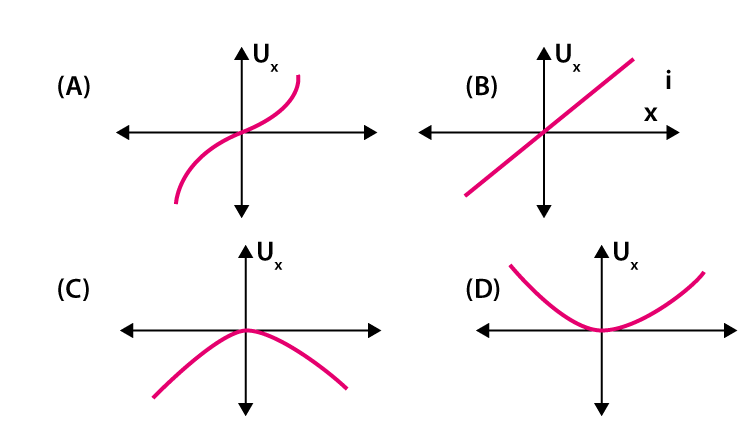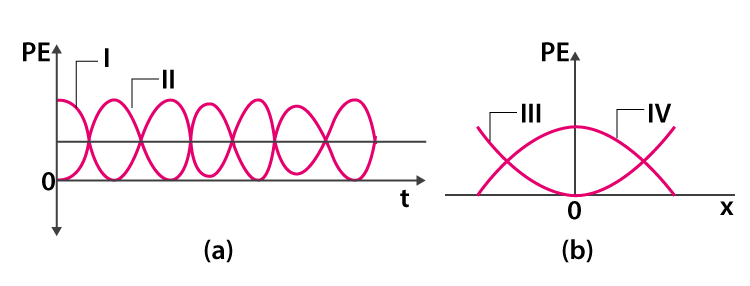# Oscillations and Waves MCQs for NEET

Oscillation is defined as the periodic fluctuation. The back and forth movement of the clock’s pendulum is an example of oscillation. The oscillator is a device in which oscillation is observed. The potential energy of the pendulum is converted into kinetic energy. When the pendulum reaches one of the ends, it has potential energy and is ready to fall. When the pendulum is in the middle potential energy gets converted into kinetic energy. This cycle continues causing oscillation in the pendulum. Periodic harmonic motion and simple harmonic motion are the two different types of oscillations.

Wave is defined as the disturbance caused in a medium that travels from one end to another. Mechanical waves, electromagnetic waves, and matter waves are the types of waves.

1. Which of the following graph depicts the variation in the potential energy for a particle executing SHM at the origin x = 0?2. Which of the following graph represents the variation in the potential energy as a function of time t and displacement x if the displacement of the particle is x = A cos⍵t(a)I,IV

(b) II, III

(c) I,III

(d) II,IV

3. What is the time taken by a particle executing SHM with a time period T sec from a positive extreme position to half of the amplitude?

(a) $$\frac{2T}{12}$$ sec

(b) $$\frac{T}{12}$$ sec

(c) $$\frac{6T}{12}$$ sec

(d) $$\frac{3T}{12}$$ sec

Answer: (a) $$\frac{2T}{12}$$ sec

4. On which of the following does the energy of a simple harmonic motion depend

(a) ⍵

(b) $$\frac{1}{\omega ^{2}}$$

(c) $$\frac{1}{a ^{2}}$$

(d) a2

5. Calculate the amplitude for a SHM using the equation x = 3sin2pt + 4cos3pt

(a) 3

(b) 5

(c) 4

(d) 7

6. What is the constant of propotionality of an oscillator if the damping force is directly propotional to the velocity

(a) kg.s-1

(b) kg.m.s-1

(c) kg.s

(d) kg.m.s-2

7. Choose the equation that cannot represent a plane progressive wave

(a) $$y=a\;sin\;\frac{2\pi }{\lambda }(Vt\mp x)$$

(b) y = A logx + B logx

(c) y = a sin (ωt 土 kx)

(d) $$y=a\;sin\;2\pi (\frac{t}{T}\mp \frac{x}{\lambda })$$

Answer: (b) y = A logx + B logx

8. What is the phase difference between the prongs of the tuning fork

(a) 5π

(b) π

(c) 2π

(d) 3π

9. What is the change in the phase if a wave is reflected from a denser medium

(a) 3π

(b) 0

(c) π

(d) 2π

10. The observer hears a sound from the source which is moving away from the observer who is stationary. Determine the frequency of the sound

(a) It is halved

(b) It remains the same

(c) It reaches infinity

(d) It gets doubled

11. The length of the pendulum which is displaying SHM is increased by 21%. Calculate the percentage increase in the periodic time of the pendulum.

(a) 21%

(b) 10%

(c) 11%

(d) 42%

12. Which of the following does not exhibit polarization

(a) Longitudinal wave in a gas

(b) Transverse wave in a gas

(c) Neither (a) nor (b)

(d) Both (a) and (b)

Answer: (a) Longitudinal wave in a gas